ISSN: 2689-2855
Current Issue
Volume No: 1 Issue No: 1

## Influence of Chemical Reaction on Marangoni Convective Flow of Nanoliquid in the Presence of Lorentz Forces and Thermal Radiation: A Numerical Investigation

Ghulam Rasool 1       Ting Zhang 1     Anum Shafiq 2     Hulya Durur 3

1School of Mathematical Sciences, Zhejiang University, Hangzhou 310027, PR-China

2Department of Mathematics, Preston University, Islamabad 44000, Pakistan

3Department of Computer Engineering, Faculty of Engineering, Ardahan University, Ardahan, Turkey

## Abstract

This study aims to numerically investigate the Marangoni convective flow of nanoliquid initiated by surface tension and heading towards a radiative Riga surface. The surface tension appears in the problem due to the gradients of temperature and concentration at the interface. The influence of first order chemical reaction is involved in the system with sufficient boundary conditions. Set of governing nonlinear PDEs is transformed into highly nonlinear ODEs using suitable transformations. HAM is applied for convergent series solutions. Impact of various pertinent fluid parameters on momentum, thermal and solutal boundary layers is analyzed graphically. The chemical reaction plays vital role in saturation of nanoparticles in the base fluid near the surface as well as away from it. The Lorentz forces originated by the Riga surface become powerful when the radiation parameter comes into effect. The significance of Riga plate is thus more prominent through thermal radiation. However, the magnetic effect dampens down for higher radiation parameter. Fluid parameters, Nusslt and Sherwood numbers are analyzed with detailed discussion and concluding remarks.

Received 10 Jan 2019; Accepted 01 Feb 2019; Published 08 Feb 2019;

Academic Editor:Inder Kaur, Nottingham Trent university, UK.

Checked for plagiarism: Yes

Review by:Single-blindThis is an open-access article distributed under the terms of the Creative Commons Attribution License, which permits unrestricted use, distribution, and reproduction in any medium, provided the original author and source are credited.

Competing interests

The authors have declared that no competing interests exist.

Citation:

Ghulam Rasool, Ting Zhang, Anum Shafiq, Hulya Durur (2019) Influence of Chemical Reaction on Marangoni Convective Flow of Nanoliquid in the Presence of Lorentz Forces and Thermal Radiation: A Numerical Investigation. Journal of Advances in Nanotechnology - 1(1):32-49.

## Introduction

Carlo Marangoni, an Italian scientist introduced the concept of surface tension gradients driven fluid. This surface tension is popped up in the surface due to gradients of temperature and concentration on the occurrence of a liquid to liquid or liquid to air interface. A liquid with higher surface tension attracts more liquid from a region with low surface tension that ultimately results in fluid flow away from the regions having low surface tension. The gradients of temperature and concentration are therefore, critical factors for such convections under Marangoni effect. A significant interest developed in investigation of heat and mass convection under this phenomenon for its vast applications in industries such as welding, crystals, melting of electronic beams etc. Consequently, numerous researchers contributed in this field after Marangoni. Lin et al 1 worked on the convection under Marangoni phenomena with thermal gradients and magnetic number variation. Exact solutions achieved by Aly and Ebaid 2 in their study on Marangoni convection of nanoliquids achieved significant appreciation in nanoliquid convection analysis. Mat et al. 3, Gevorgyan et al. 4 and Al-sharafi et al. 5 have also contributed in this field of study with valuable results there in.

Engineers, Scientists working in the field of Nuclear energy, and Pharmaceutics come across the problem of rise in temperature in the working machine at a high speed performance. This situation was a big reason to worry in fluid mechanics before the introduction of Nanoliquids. The Idea of nanoliquid was introduced by Choi 6 in his research study. Pouring nanoparticles in a base fluid of poor conductivity showed drastically efficient results in the aspect of thermal conductivity of the base fluid. These impurities improved the conductivity of the fluid up to a significant level and the problem of machine heating was controlled with improved efficiency. Later on, Ibanez et al. 7 studied MHD nanoliquids analytically assuming convective boundary conditions. Hayat et al. 8 studied flow on stagnation point with an inclined magnetic field considering a nanofluid. Anum et al. 9 analyzed a third grade nanoliquid flow over a Riga plate with Cattaneo-Christov model in application. Hayat et al. 10 studied MHD-Powell-Eyring nanofluid flow with convective conditions. Alsabery et al. 11 studied heat flux in simulating the nanoliquid obtaining good results in Nusslt number. Numerous applications have been presented by Sheikholeslami and Ganji 12 in their research for useful nanoliquids. Nasrin et al. 13 studied free convection in aspect of nanoliquid passing through a chamber. Williamson nanoliquid has been analyzed by Bhatti and Rashidi 14. Parvin et al. 15 studied free convection through curved cavity using water based nanoliquid. Selimefendigil and Oztop 16 studied conjugate convection via titled cavity. Reddy et al. 17 performed numerical simulations of the mixed convection using two phase fluid model through a plate. For more related works one can see 18, 19, 20, 21, 22, 23, 24, 25, 26, 27, 28, 29, 30.

Fluid flow analysis in the field of fluid mechanics has always been dependent on various external influencing agents. Researchers working in the field of Astrology and Geo physics always need such kind of external agents to ease the movement of fluid in their processing. Most of the fluids for example plasma are typically dependent on the magnetic induction for their flow phenomena. Reason of this dependence of fluids on external agents is poor conductivity of fluids. The problem was somehow reduced with the introduction of Riga plate, an array of permanently mounted magnets and alternating electrodes as displayed in the model of this paper. Gailitis and Lielausis 31 introduced this array in their study for the first time which is treated as a hallmark in the field of fluid mechanics. Later on, Ahmed et al. 32 studied the impacts of zero mass flux on fluids involving Riga plate in their model. Sheikholeslami et al. 33 in their research concluded that temperature profile shows decreasing behavior with strong Marangoni number (r) due to Lorentz forces. Shafiq et al. 34 studied fluid point-flow based Walters-B model involving a Riga plate in their study and found fruitful results in the aspect of thermal characteristics. Adeel et al. 35 analyzed mixed convection nanoliquids mounting a vertical Riga plate in the way of fluid flow with strong suction.

Numerous articles on nanoliquids are available in the literature in the context of heat and mass flux with different variables and different systematic approaches. However, the use of Riga plate for generation of magnetic effect together with effect of chemical reaction is not found in the literature as for as to the knowledge of the author that assures the novelty of this research work. In this study, firstly we have involved the Riga plate to generate Lorentz forces in the system. The chemical reaction effect and thermal radiation effect are considered. Secondly, the set of PDEs is converted into set of nonlinear ODEs with transformations using the technique of non-dimensionalization 36. The non-dimensionalized system is subjected to HAM 37, 38, 39, 40, 41, 42 for convergent series solutions. Thirdly, the convergence of the results is analyzed graphically and finally, the results are plotted with sufficient discussion on the behavior of flow profiles.

## Mathematical Model

A two dimensional steady and in-compressible nanoliquid is considered under Marangoni effect heading towards a radiative Riga surface. The flow is driven by tension appearing in the surface due to the temperature and concentration gradients. Thermal radiation and chemical reaction effects are utilized. The Brownian motion factor and Thermphoresis phenomena are of significant importance in this study. The temperature Tfrelates the temperature gradient whereas Cf relates the concentration gradient with base fluid. The heat-mass flux is considered along x-axis in Cartesian coordinates. Figure 1 displays the physical scenario for the aforementioned problem. The governing equations are therefore:

The surface tension s, being a function of T and C can be defined as follows: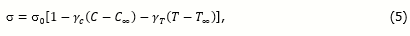where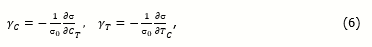with following boundary conditions,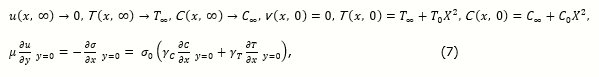Figure 1. Schematic Diagram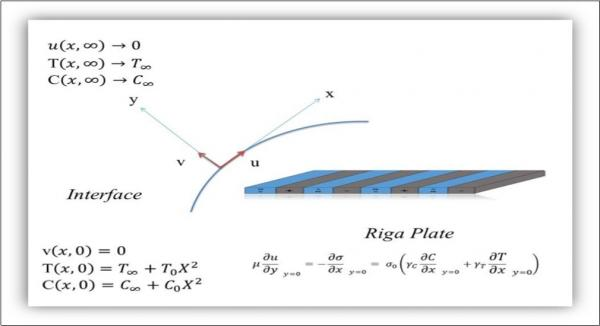Here u, v represent the velocity components in x, y directions, respectively. μ is symbol of dynamic viscosity, v is the kinematic viscosity, ρf represents the density of fluid, K is used for chemical reaction, σ the surface tension, T0 and C0 are temperature and concentration on the surface, α is representing thermal diffusivity of the fluid, k is symbol of thermal conductivity of fluid. 𝜏 is used for ratio between heat capacity of the fluid, k is symbol of thermal conductivity of fluid. 𝜏 is used for ratio between heat capacity of the nanoparticles (PC)p and heat capacity of base fluid, (PC)f , DB is Brownian diffusion, DT is Thermophoresis, and qr is the typical radiative heat flux that can be written through Rosseland's approximation as follows: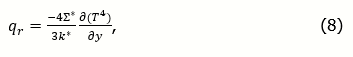where ∑* and K*are Stefan-Boltzmann's constant and coefficient of mean absorption, respectively. Using Taylor's series and omitting second and higher order terms, we get,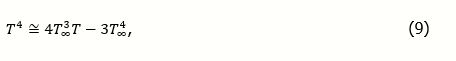which upon substitution in (8) yields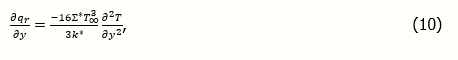Equation (10) in (3) gives,

Define,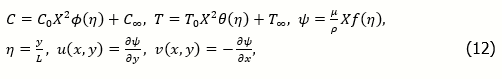We obtain,

With,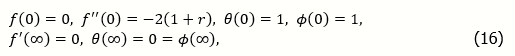where 𝜸 = (Co𝜸C) / (To𝜸T) is the ratio of thermal to solutal surface tension s.t. R=((C0-C)γC)/((T0-T)γT) and Ma|L,T=(L𝝙T𝜸T|C)/ να , Ma|L,C=(L𝝙CγC|T)/να are thermal and solutal Marangoni numbers resulting 𝜸=Ma\L,T / Ma\L,C is the Marangoni ratio. (Q=L4πj0 M0/8ν2xρ) is the modified Hartman number, (Pr = ν/α) is the Prandtl number, (Nb=(ρc)p DB C0 x2/ (ρc)f L2 a) is the Brownian motion factor, Sc=ν/D is the Schmidt number,(Nt= (ρc)p DT x2) / (ρc)f L2 a ) is Thermophoretic factor, (Rd= 4Σ* T3/ kk*), is radiation parameter and, (β=πL/a) is dimensionless parameter. Skin friction coefficient, local Nusselt and Sherwood numbers are defined by,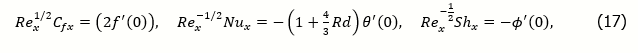where Rex = ux/v represents the local Reynolds number.

## Numerical Simulation

The efficiency of Homotopy analysis method (HAM) for solving non-linear ODEs has been witnessed through literature. Researchers have given preference to this technique over various other famous methods. The method starts with assumption of some suitable initial guess subject to the boundary conditions given in the problem. Let,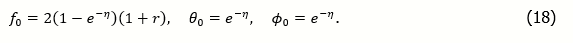One can see that (16) is satisfied. Define,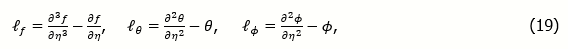such that,

Where ai are constants for. i = 1-7.The 0thorder deformation problems are: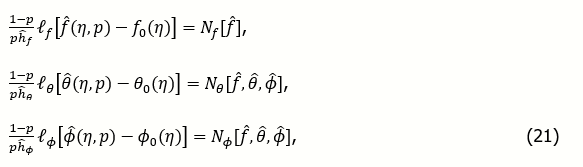subject to,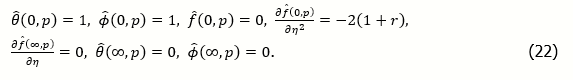Resulting the following system,

where p[0,1] is a typical embedding parameter and ĥf, ĥθ, ĥϕ are so-called auxiliary parameters with Nf, Nθ, Nϕ are the non-linear operators. For p=0,1, we have: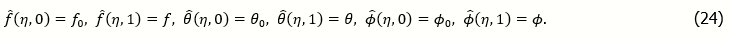Using Taylor's expansion,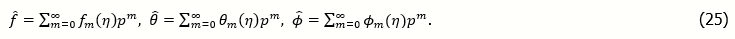The convergence of (24) is purely dependent on the choice of ĥ. For p such that the system (24) converges, we write,

The mth order deformation problems are,

where ϕm=1for m>1 otherwise 0. Finally,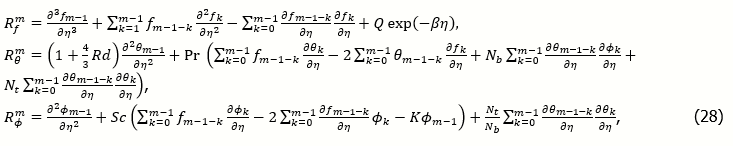with following general solutions to the problem,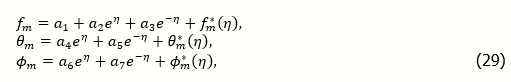where ai are constants for i =1-7 and fm*(η), θm*(η),ϕm*(η)are special solutions.

## Convergence of Solutions

The auxiliary parameters used for the flow profiles in series solutions in HAM are typically considered for controlling the convergence. These parameters significantly moderate the convergence rates thus are vital in achieving the convergence of final solutions. Convergence interval of f, θ and ϕ are sketched in Figure 2. One can see that the intervals of convergence for the aforementioned three profiles are (-0.40, 0.05), (-0.45, 0.05) and (-0.45, 0.05), respectively. The solutions show convergence after19th iteration for velocity profile and 26th iteration for temperature and concentration profiles, respectively.

Figure 2. H-Curves for Velocity, Temperature and Concentration Profiles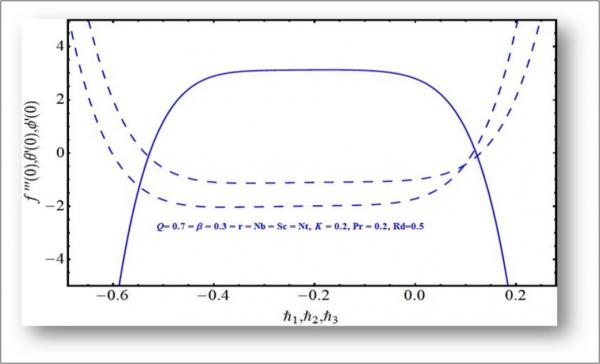## Results and Discussion

We examine the behavior of a surface tension driven nanofluid under the action of Lorentz forces generated by Riga surface and the chemical reaction inside the fluid. The flow is assumed in two dimensions such that x-axis is parallel to the fluid flow and y-axis is normal to the surface of the Riga plate. Influence of pertinent fluid parameters on flow profiles is plotted graphically and the discussion on these graphs is as follows. Figure 3 presents the behavior of velocity profile with variation in the dimensionless parameter β. The elevated values of β enhance the fluid viscosity that results in decreasing the flow momentum and corresponding boundary layer drops down. The flow velocity receives enhancement with elevated values of modified Hartman number as displayed in Figure 4. The graph of f(η) shows augmented variation with augmented values of. Q The induced Lorentz forces parallel to the flow profile enhance the surface tension produced in fluid that certainly drives the fluid with stronger force. Consequently the flow profile receives an increasing behavior. Figure 5 shows the behavior of velocity profile and associated boundary layer with variation in Marangoni ratio (r). One can see an increasing behavior in profile for elevated values of r. Figure 6 is the display of temperature profile against the dimensionless parameter β. The temperature drops down for elevated values of β. The rise in viscosity for elevated values of β results in slow motion of fluid and consequently lessens the collisions in between fluid particles as well as between fluid particles and nanoparticles that results in decreasing behavior of temperature profile. The same is noticed with augmented values of Q plotted in Figure 7. The temperature profile and associated boundary layer shows a decreasing behavior. A significantly prominent increase in temperature profile is witnessed for larger values of Prandtl number (Pr) portrayed in Figure 8. Since, Prandtl number is the ratio of momentum diffusivity to thermal diffiusivity, therefore, the elevated values of P𝜸 number enhance the momentum diffusivisity that results in enhancement of temperature profile and associated boundary layer. Not prominent, but comparatively an increasing behavior is noticed in temperature profile with enhancement in Thermophoretic factor (Nt) as plotted in Figure 9. The enhancement is dependent on the strong Thermophoretic force that results the away

Figure 3. Velocity profile against ß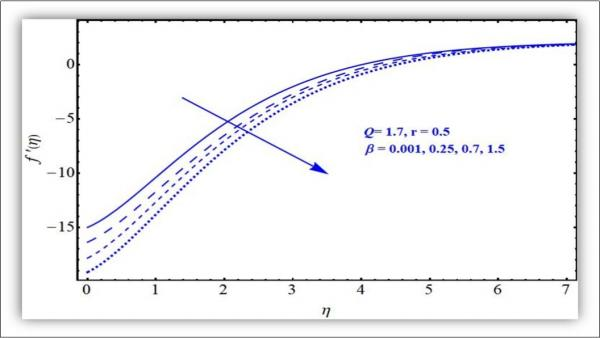Figure 4. Velocity profile against Q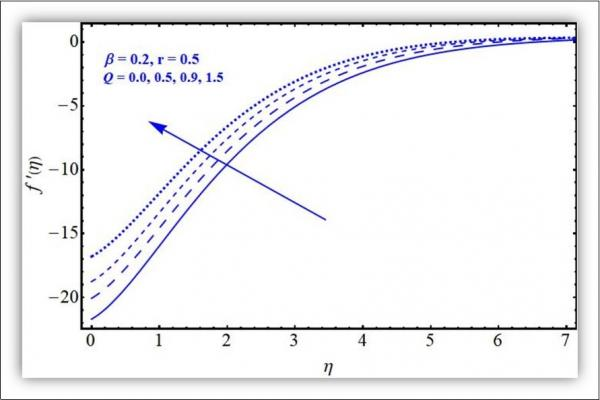Figure 5. Velocity Profile against r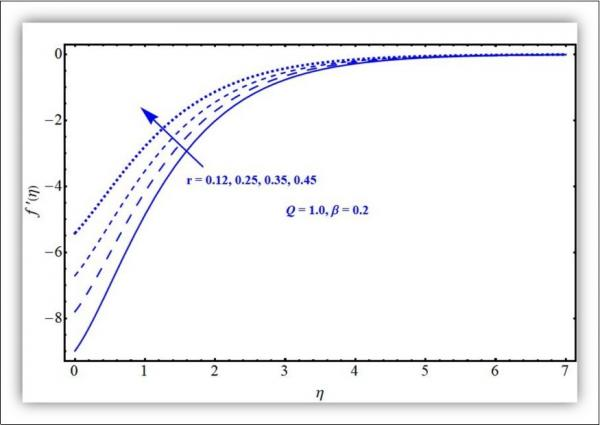Figure 6. Temperature profile against ß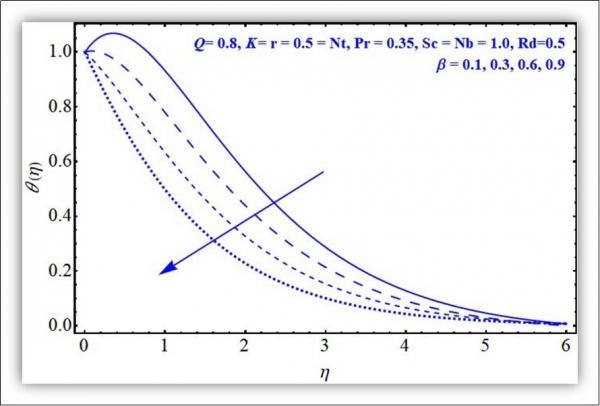Figure 7. Temperature profile against Q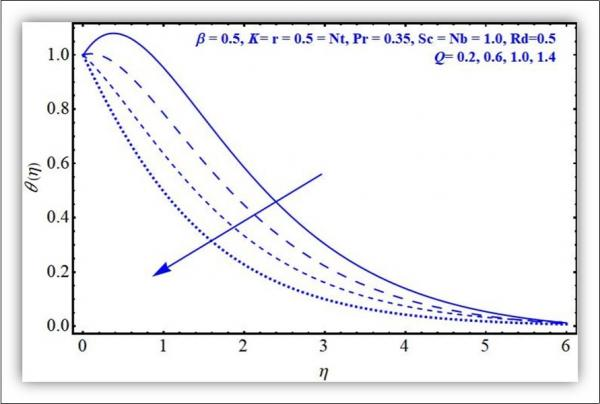Figure 8. Temperature profile against Pr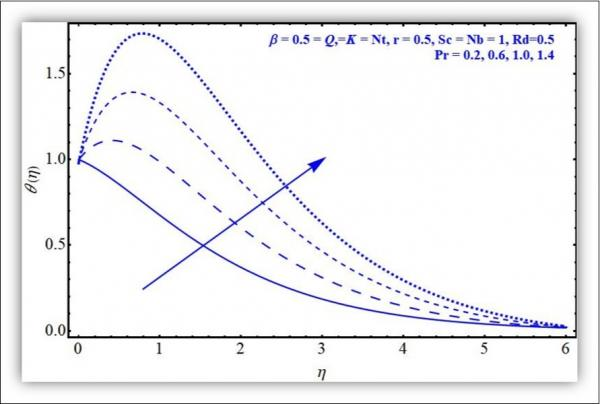Figure 9. Temperature profile against Nt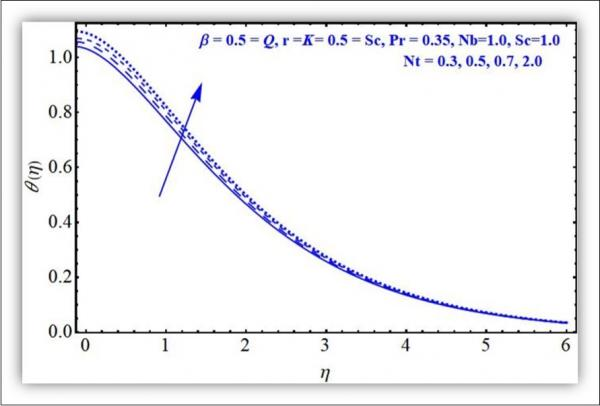movement of nanoparticles from the surface of Riga. Figure 10 is the display of influence of radiation factor (Rd) on the temperature profile. Temperature profile receives prominent enhancement in its behavior with elevated values of radiation factor. Thus the addition of radiation factor enhances the heat flux up to a significant level. Figure 11 is plotted to analyze the behavior of concentration of nanoparticles against the variation in Brownian motion factor (Nb). The stronger Brownian motion factor enhances the in-predictive motion of fluid particles that results in enhancement of temperature distribution. This enhancement in temperature drops down the concentration of nanoparticles near the Riga surface. The concentration profile enhances with elevated values of Schmidt number (Sc) for certain reasons as shown in Figure 12. The main reason is the enhancement in Brownian diffusivity that results in enhancement of associated boundary layer of concentration of nanoparticles in the base fluid. Elevated values of both the chemical reaction and radiation factor result in decreasing behavior of the concentration profile displayed in Figure 13 and Figure 14, respectively. The enhancement in chemical reaction results in away movement of nanoparticles from the surface that ultimately effects the concentration in the base fluid. The elevated values of Thermophoretic factor (Nt) result in enhancement of heat flux but drops down the mass flux while the enhancement in Prandtl results in decreasing behavior of the Sherwood number (the mass flux) as plotted in Figure 15, Figure 16 and Figure 17.

Figure 10. Temperature profile against Rd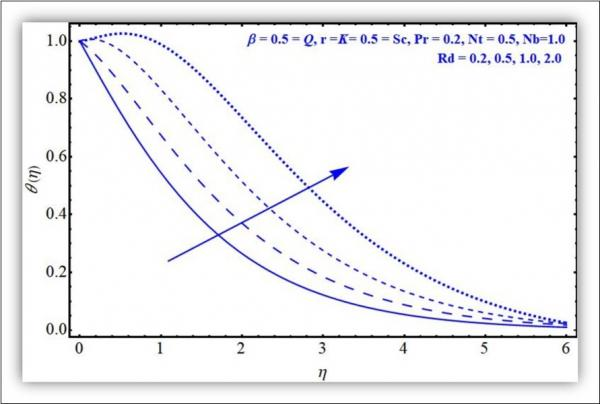Figure 11. Concentration profile against Nb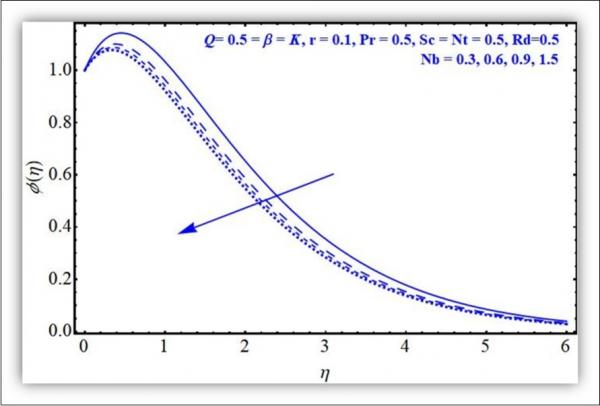Figure 12. Concentration profile against Sc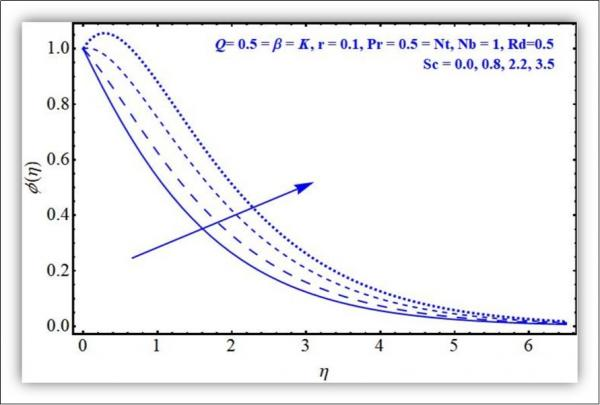Figure 13. Concentration profile against K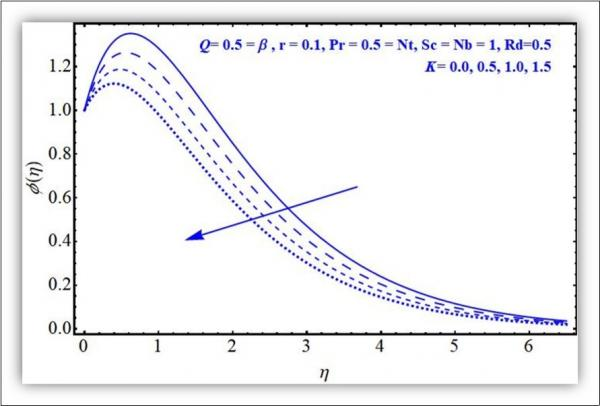Figure 14. Concentration profile against Rd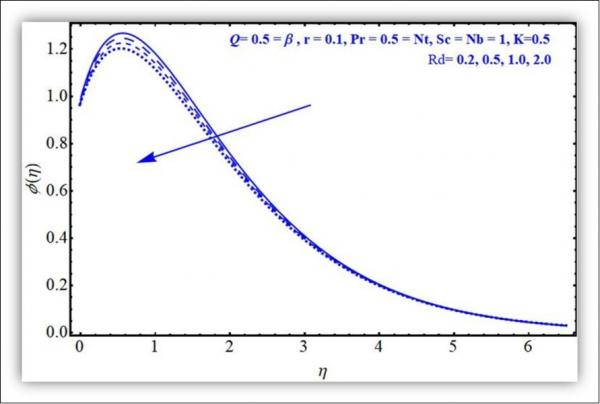Figure 15. Nusslt number against Nb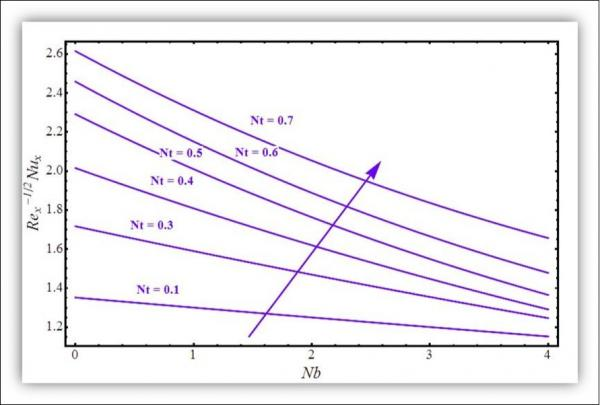Figure 16. Sherwood number against Nb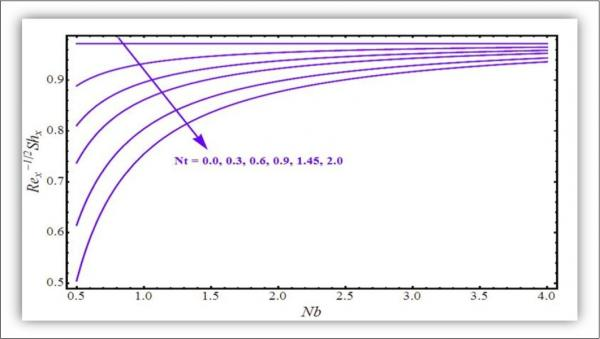Figure 17. Sherwood number against Nb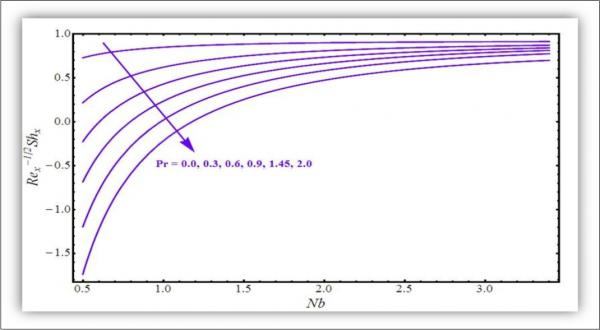## Comparison

This subsection summarizes the result with a precise comparison of present results with 33. Setting M=0 in 33 and Q=0=K=Rd in present model, the left over system of equations and the graphical results are exactly the same in either case as presented in Figure 18 for the velocity profile against Marangoni ratio (r). However, considering the effects of Q, K and Rd, we observe that there is a significant variation in the graphical results in the present work as compared to the previous work. For example, the elevated values of 𝜸 result in a rapid increasing behavior of velocity profile in the present case as compared to 33 confirming a more prominent effect of Marangoni ratio (r) on the flow profiles.

Figure 18. Comparison with 33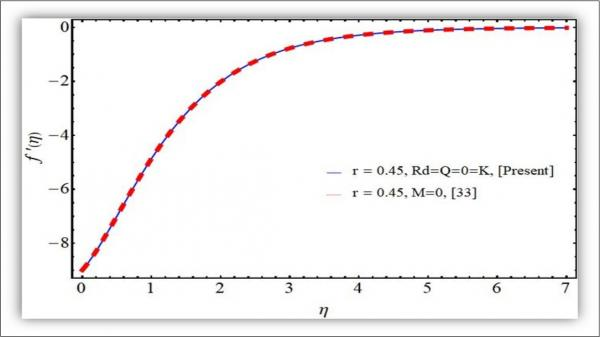## Closing Remarks

We examine the behavior of a surface tension driven nanofluid under the action of Lorentz forces generated by Riga surface and the chemical reaction inside the fluid. The flow is assumed in two dimensions such that x-axis is parallel to the fluid flow and y-axis is normal to the surface of the Riga plate. The final governing equations after application of suitable transformations with sufficient boundary conditions have been solved by HAM. The convergent series solutions are presented and analyzed graphically. Following are salient conclusions

Velocity profiles receives prominent enhancement with stronger Marangoni factor (r) however, the elevated values of dimensionless parameter β result in decreasing behavior of flow velocity and the associated boundary layer drops down.

Prandtl number is an enhancing factor for the temperature distribution.

Both the radiation and thermophoresis are increasing factors of temperature distribution.

Chemical reaction forces the away movement of nanoparticles from Riga surface. A prominent decreasing behavior is witnessed with elevated values of K.

Heat flux enhances with augmented values of Thermophoretic factor (Nt).

Mass flux declines with augmented values of Thermophoretic factor (Nt) and the Prandtl factor (Pr).

## Cited by (9)

1.Ansari Md Sharifuddin, Magagula Vusi Mpendulo, Trivedi Mumukshu, 2020, Jeffrey nanofluid flow near a Riga plate: Spectral quasilinearization approach, Heat Transfer, 49(3), 1491, 10.1002/htj.21673
2.Shafiq Anum, Zari Islam, Rasool Ghulam, Tlili Iskander, Khan Tahir Saeed, 2019, On the MHD Casson Axisymmetric Marangoni Forced Convective Flow of Nanofluids, Mathematics, 7(11), 1087, 10.3390/math7111087
3.Lund Liaquat Ali, Omar Zurni, Khan Ilyas, Raza Jawad, Sherif El-Sayed M., et al, 2020, Magnetohydrodynamic (MHD) Flow of Micropolar Fluid with Effects of Viscous Dissipation and Joule Heating Over an Exponential Shrinking Sheet: Triple Solutions and Stability Analysis, Symmetry, 12(1), 142, 10.3390/sym12010142
4.Oyelakin Ibukun Sarah, Sibanda P., 2020, Homogeneous–heterogeneous reactions in flow past a horizontal circular cylinder with an induced magnetic field and nonlinear thermal radiation, Heat Transfer, 49(2), 1065, 10.1002/htj.21653
5.Shafiq Anum, Khan Ilyas, Rasool Ghulam, Seikh Asiful H., Sherif El-Sayed M., 2019, Significance of Double Stratification in Stagnation Point Flow of Third-Grade Fluid towards a Radiative Stretching Cylinder, Mathematics, 7(11), 1103, 10.3390/math7111103
6.Shafiq Anum, Rasool Ghulam, Khalique Chaudry Masood, 2020, Significance of Thermal Slip and Convective Boundary Conditions in Three Dimensional Rotating Darcy-Forchheimer Nanofluid Flow, Symmetry, 12(5), 741, 10.3390/sym12050741
7.Shafiq Anum, Khan Ilyas, Rasool Ghulam, Sherif El-Sayed M., Sheikh Asiful H., 2020, Influence of Single- and Multi-Wall Carbon Nanotubes on Magnetohydrodynamic Stagnation Point Nanofluid Flow over Variable Thicker Surface with Concave and Convex Effects, Mathematics, 8(1), 104, 10.3390/math8010104
8.Ibrahim Wubshet, Zemedu Chaluma, 2020, Numerical solution of micropolar nanofluids with Soret, Dufor effects and multiple slip conditions, Journal of Physics Communications, 4(1), 015016, 10.1088/2399-6528/ab5260
9.Rasool Ghulam, Zhang Ting, Chamkha Ali J., Shafiq Anum, Tlili Iskander, et al, 2019, Entropy Generation and Consequences of Binary Chemical Reaction on MHD Darcy–Forchheimer Williamson Nanofluid Flow Over Non-Linearly Stretching Surface, Entropy, 22(1), 18, 10.3390/e22010018